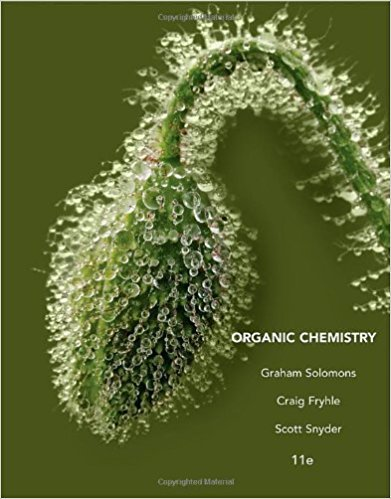×
×

# Solutions for Chapter 19: Condensation and Conjugate Addition Reactions of Carbonyl Compounds## Full solutions for Organic Chemistry | 11th Edition

ISBN: 9781118133576Solutions for Chapter 19: Condensation and Conjugate Addition Reactions of Carbonyl Compounds

Solutions for Chapter 19
4 5 0 383 Reviews
28
3
##### ISBN: 9781118133576

Organic Chemistry was written by and is associated to the ISBN: 9781118133576. This textbook survival guide was created for the textbook: Organic Chemistry, edition: 11. Chapter 19: Condensation and Conjugate Addition Reactions of Carbonyl Compounds includes 56 full step-by-step solutions. This expansive textbook survival guide covers the following chapters and their solutions. Since 56 problems in chapter 19: Condensation and Conjugate Addition Reactions of Carbonyl Compounds have been answered, more than 47222 students have viewed full step-by-step solutions from this chapter.

Key Chemistry Terms and definitions covered in this textbook
• bomb calorimeter

A device for measuring the heat evolved in the combustion of a substance under constant-volume conditions. (Section 5.5)

• bond angles

The angles made by the lines joining the nuclei of the atoms in a molecule. (Section 9.1)

• calorimeter

An apparatus that measures the heat released or absorbed in a chemical or physical process. (Section 5.5)

• Carbanion

An ion in which carbon has an unshared pair of electrons and bears a negative charge.

• Claisen condensation

A nucleophilic acyl substitution reaction in which the nucleophile is an ester enolate and the electrophile is an ester.

• complete ionic equation

A chemical equation in which dissolved strong electrolytes (such as dissolved ionic compounds) are written as separate ions. (Section 4.2)

• conversion factor

A ratio relating the same quantity in two systems of units that is used to convert the units of measurement. (Section 1.6)

• crystallite

A region of a polymer inwhich the chains are linearly extended and closein proximity to one another, resulting in van der Waals forces that hold the chains close together.

• cyanides.

Compounds containing the CN2 ion. (22.3)

• Dihedral angle

The angle created by two intersecting planes.

• dipole moment (m)

The amount of partial charge (d ) on either end of a dipole multiplied by the distance of separation (d): m=d × d

• Disulfide

A molecule containing an !S!S! group

• divalent

An element that forms two bonds, such as oxygen.

• Double helix

A type of secondary structure of DNA molecules in which two anti parallel polynucleotide strands are coiled in a right-handed manner about the same axis

• electron

A negatively charged subatomic particle found outside the atomic nucleus; it is a part of all atoms. An electron has a mass 1>1836 times that of a proton. (Section 2.3)

• periodic table

The arrangement of elements in order of increasing atomic number, with elements having similar properties placed in vertical columns. (Section 2.5)

• photoionization

The removal of an electron from an atom or molecule by absorption of light. (Section 18.2)

• pressure

A measure of the force exerted on a unit area. In chemistry, pressure is often expressed in units of atmospheres (atm) or torr: 760 torr = 1 atm; in SI units pressure is expressed in pascals (Pa). (Section 10.2)

• Resolution

Separation of a racemic mixture into its enantiomers; in mass spectrometry, a measure of how well a mass spectrometer separates ions of different mass.

• Sulfi de

The sulfur analog of an ether; a molecule containing a sulfur atom bonded to two carbon atoms. Sulfi des are also called thioethers

×